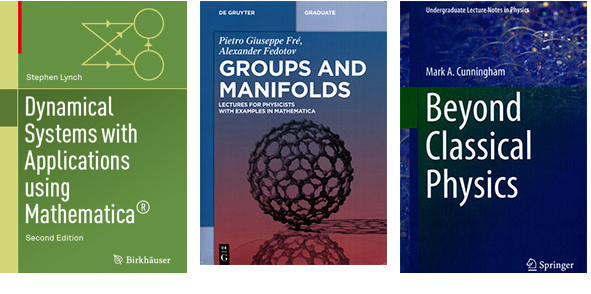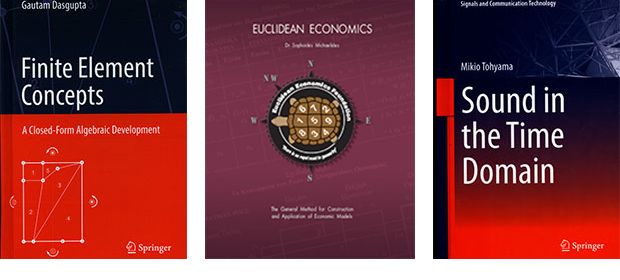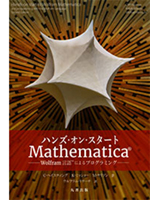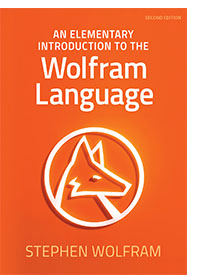Browse by Topic
Related Topics

# Language upon Language: New Wolfram Language–Based Book Releases

Here at Wolfram Research, we’re always looking to add fresh material to our reading lists, and this winter brings a crop of new books that make use of the Wolfram Language’s power and versatility. Physics and math are represented, as usual, but economics and specialized financial mathematics make a showing as well. Also of note, a musician and engineer analyzes “sound in the time domain.” Brilliant minds prove once again that, with the Wolfram Language, the possibilities are endless.## Dynamical Systems with Applications Using Mathematica, Second Edition

Author Stephen Lynch provides an introduction to the theory of dynamical systems. With the aid of Mathematica, this book’s hands-on approach first deals with continuous systems using ordinary differential equations, while the second part is devoted to the study of discrete dynamical systems. Lynch takes the reader from basic theory to recently published research material. Emphasized throughout are numerous applications to biology, chemical kinetics, economics, electronics, epidemiology, nonlinear optics, mechanics, population dynamics and neural networks.

## Groups and Manifolds: Lectures for Physicists with Examples in Mathematica

Groups and Manifolds is an introduction to the mathematics of symmetry, with a variety of examples for physicists. Authors Pietro Giuseppe Frè and Alexander Fedotov cover both classical symmetry—as seen in crystallography—and the mathematical concepts used in supersymmetric field theories. After a basic introduction to group theory, they discuss Lie algebras and basic notions of differential geometry. Mathematica allows readers to develop group-theoretical constructions.

## Beyond Classical Physics

In this undergraduate textbook, Mark A. Cunningham discusses the nature of the microscopic universe from a modern perspective, based on Einstein’s notions of relativity and Noether’s proof of the emergence of conservation laws from symmetries of the equations of motion. These ideas drove the development of the Standard Model of particle physics and subsequent attempts to define a unified (string) theory. The second half of the book explores various aspects of many-body physics, ranging from chemical systems to plasmas to black holes. Cunningham makes extensive use of Mathematica to enable students to explore the meanings of different equations in a graphical manner. Students will gain an appreciation of the current state of physical theory in preparation for more detailed, advanced study as upperclassmen.## Finite Element Concepts: A Closed-Form Algebraic Development

Author Gautam Dasgupta presents a highly original treatment of the fundamentals of FEM, developed using computer algebra, based on undergraduate-level engineering mathematics and the mechanics of solids. The book is divided into two distinct parts of nine chapters and seven appendices. The appendices give a short introduction to Mathematica, followed by truss analysis using symbolic codes that could be potentially used in all FEM problems to assemble element matrices and solve for all unknowns. All Mathematica codes for theoretical formulations and graphics are included, with extensive numerical examples.

## Euclidean Economics

Originally written in Greek, Euclidean Economics is now updated and translated to English. Author Sophocles Michaelides contends that economics can be studied and utilized on the basis of a minimum of fundamental hypotheses and the general laws of mathematics. He uses the Wolfram Language exclusively in the book’s calculations. Part two of the book includes all the notebooks in printed form.

## Sound in the Time Domain

Author Mikio Tohyama is a engineer who plays the piano every day, so he is attuned to sound on both scientific and experiential levels. He uses the Wolfram Language extensively to generate figures illustrating the nature of sound, focusing on the characteristics of sound waves in the context of time structures. This time-domain approach provides an informative and intuitively understandable description of various acoustic topics, such as sound waves traveling in an acoustic tube or in other media where spectral or modal analysis can be intensively performed.

## Hands-on Start to Wolfram Mathematica with the Wolfram Language

### Second Edition (in Japanese)Authors Cliff Hastings, Kelvin Mischo and Michael Morrison have updated the book hailed as “a much-needed compliment to existing support material for Mathematica… that puts to rest, once and for all, the claim that Mathematica is difficult and not accessible to the school and college levels of our computational universe” (Fred Szabo at Concordia University in Montreal, author of Actuaries’ Survival Guide). And now this second edition of the definitive guide to the Wolfram Language is available in Japanese.

## An Elementary Introduction to the Wolfram Language

Stephen Wolfram’s An Elementary Introduction to the Wolfram Language, now in its second edition, remains the premium gateway for anyone interested in learning programming and computational thinking through the Wolfram Language.While available in print from all major booksellers, the complete text is also available free online, and forms the basis of a newly launched fully interactive course through Wolfram U. Wolfram U expands educational opportunities with free courses and video classes on everything from data science and statistics to machine learning and image processing. Teachers seeking to incorporate coding in the classroom should also consider Stephen Wolfram’s blog post, “Machine Learning for Middle Schoolers,” which includes an overview of the book.

An Elementary Introduction to the Wolfram Language is available in English, Chinese and Korean, and is coming soon in Spanish and Russian.